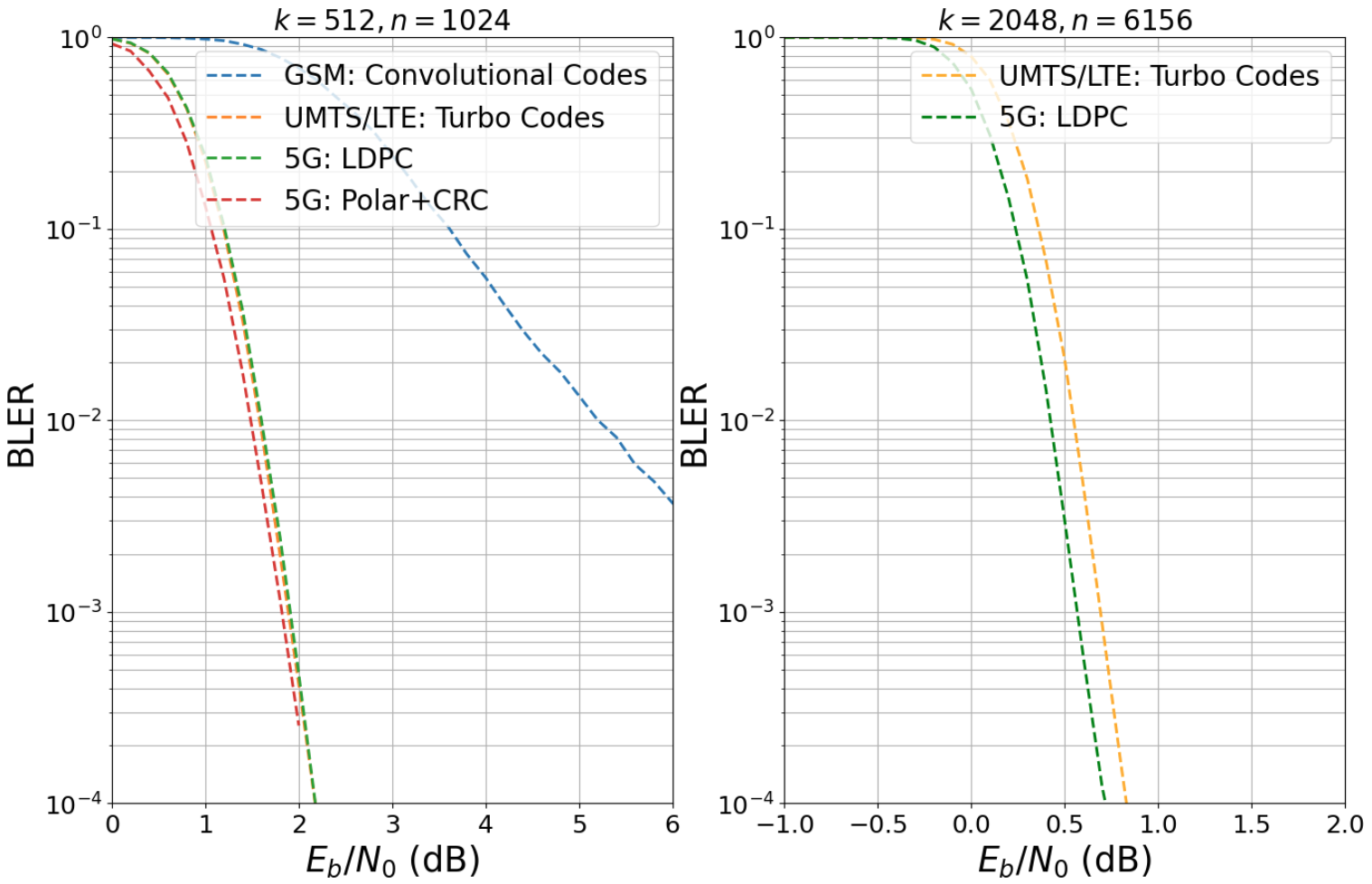# Forward Error Correction (FEC)

The forward error correction (FEC) package provides encoding and decoding algorithms for several coding schemes such as low-density parity-check (LDPC), Polar, Turbo, and convolutional codes as well as cyclic redundancy checks (CRC).

Although LDPC and Polar codes are 5G compliant, the decoding algorithms are mostly general and can be used in combination with other code designs.

Besides the encoding/decoding algorithms, this package also provides interleavers, scramblers, and rate-matching for seamless integration of the FEC package into the remaining physical layer processing chain.

The following figure shows the evolution of FEC codes from GSM (2G) up to the 5G NR wireless communication standard. The different codes are simulated with the Sionna FEC package for two different codeword length of $$n=1024$$ (coderate $$r=1/2$$) and $$n=6156$$ (coderate $$r=1/3$$), respectively.

Remark: The performance of different coding scheme varies significantly with the choice of the exact code and decoding parameters which can be found in the notebook From GSM to 5G - The Evolution of Forward Error Correction. Further, the situation also changes for short length codes and results can be found in 5G Channel Coding: Polar vs. LDPC Codes.Please note that the best choice of a coding scheme for a specific application depends on many other criteria than just its error rate performance:

• Decoding complexity, latency, and scalability

• Level of parallelism of the decoding algorithm and memory access patterns

• Error-floor behavior